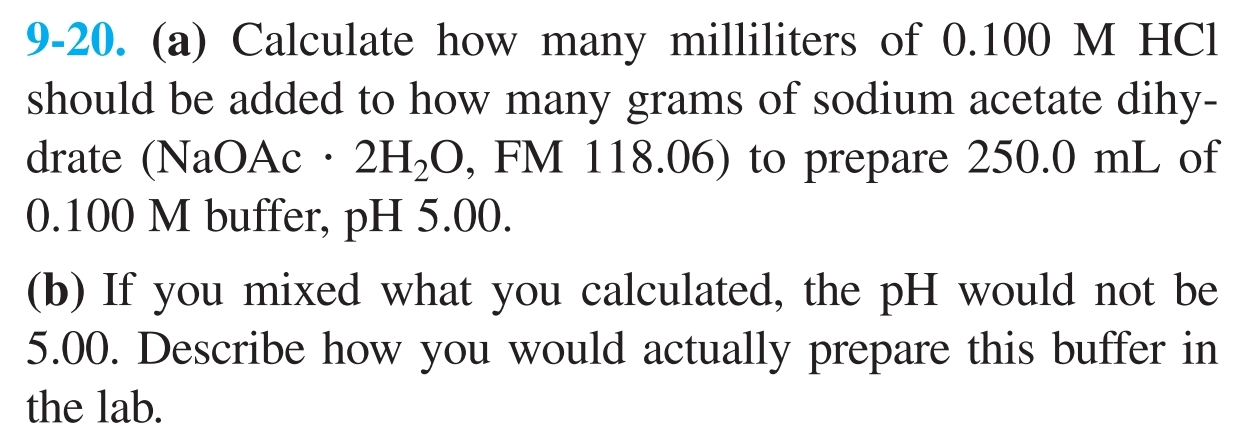# 9-20. (a) Calculate how many milliliters of 0.100 M HCIshould be added to how many grams of sodium acetate dihy-drate (NaOAc· 2H2O, FM 118.06) to prepare 250.0 mL of0.100 M buffer, pH 5.00.(b) If you mixed what you calculated, the pH would not be5.00. Describe how you would actually prepare this buffer inthe lab.

Question
139 viewshelp_outlineImage Transcriptionclose9-20. (a) Calculate how many milliliters of 0.100 M HCI should be added to how many grams of sodium acetate dihy- drate (NaOAc· 2H2O, FM 118.06) to prepare 250.0 mL of 0.100 M buffer, pH 5.00. (b) If you mixed what you calculated, the pH would not be 5.00. Describe how you would actually prepare this buffer in the lab. fullscreen
check_circle

Step 1

As the volume of the buffer solution is 250 mL, the volume of HCl solution to be added will be 250 mL assuming the addition of sodium acetate does not affect the volume of the solution. The number of moles of HCl present (nHCl) in 250 mL 0.1 M solution is calculated using equation (1) in which n is the number of moles, C is the concentration and V is the volume of solution.

Step 2

Consider ‘x’ be the mass of sodium acetate to be added. So, the number of moles of sodium acetate (nNaOAc) is calculated using equation (2) in which n is the number of moles, m is the mass of the substance and M is the molar mass of the substance.

Step 3

As HCl reacts with NaOAc to produce acetic acid (AcOH). 0.025 mol of HCl will react with 0.025 mol of NaOAc to produce 0.025 mol of Ac...

### Want to see the full answer?

See Solution

#### Want to see this answer and more?

Solutions are written by subject experts who are available 24/7. Questions are typically answered within 1 hour.*

See Solution
*Response times may vary by subject and question.
Tagged in

### Equilibrium Concepts